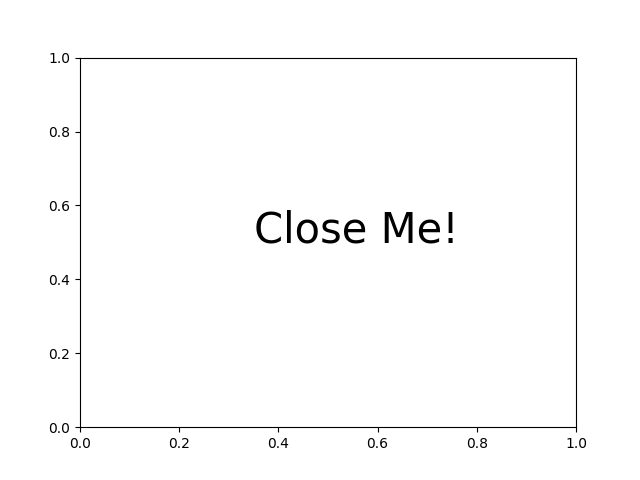Close Event¶

Example to show connecting events that occur when the figure closes.import matplotlib.pyplot as plt

def on_close(event):
print('Closed Figure!')

fig = plt.figure()
fig.canvas.mpl_connect('close_event', on_close)

plt.text(0.35, 0.5, 'Close Me!', dict(size=30))
plt.show()

Keywords: matplotlib code example, codex, python plot, pyplot Gallery generated by Sphinx-Gallery